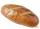Coal storage

The coal storage distribute received coal shipment within three days. The first day distribute third of the shipments, the second day of two-fifths of the rest and the third day 300 tons of coal. How many tons of coal distributed first and second day?

Result

a =  250 t
b =  200 t

Solution:

a+b+300 = t
a = t/3
b = 2/5(t - a)

a+b-t = -300
3a-t = 0
2a+5b-2t = 0

a = 250
b = 200
t = 750

Calculated by our linear equations calculator.

Leave us a comment of this math problem and its solution (i.e. if it is still somewhat unclear...):Be the first to comment!To solve this verbal math problem are needed these knowledge from mathematics:

Need help calculate sum, simplify or multiply fractions? Try our fraction calculator. Do you have a linear equation or system of equations and looking for its solution? Or do you have quadratic equation?

Next similar math problems:

1. ClassroomOne-eighth of 9th class was interested in studying at a grammar school, at a business academy one sixth, at secondary vocational schools quarter, to SOU one third and the remaining three students were interested in the school of art direction. How many st5 of the same bread has the same weight as three bread and 4 kg of fruit. What weight has one bread?
3. Eq-fracSolve the following equation with fractions: h + 1/3 =5/3
4. Fraction + eqSolve following simple equation with fractions: -5/6(8+5b) = 75 + 5/3b
5. Simple equation 8Solve the following equation: 36=-(1+7x)-6(-7-x)
6. Fraction to decimalWrite the fraction 3/22 as a decimal.
7. Lengths of the poolMiguel swam 6 lengths of the pool. Mat swam 3 times as far as Miguel. Lionel swam 1/3 as far as Miguel. How many lengths did mat swim?
8. One thirdIf 3/5 is 360, how much is 1/3?
9. Equation 29Solve next equation: 2 ( 2x + 3 ) = 8 ( 1 - x) -5 ( x -2 )
10. The bunsKate, Zofia and Peter Liked buns. Even today, their grandmother prepare their favorite meal. Katka eats 4 bunches, Žofia 3 and Petra eats 5 buns. Their grandmother said to them, "My inmate will you know how many buns I have been make today, if those you ea
11. Simple equationSolve for x: 3(x + 2) = x - 18
12. Grandmother and grandfatherGrandmother baked cakes. Grandfather ate half, then quarter of the rest ate Peter and Paul ate half of rest. For parents left 6 cakes. How many cakes maked the grandmother?
13. Equation 20In given equation: 8/9-4/5=2/9+x, find x
14. Unknown numberI think the number. I'll reduce it to its one-third. The result is then increased by one-third, and I get the number 12.
15. Missing numberBlank +1/6 =3/2 find the missing number
16. FruitsAmy bought a basket of fruits 1/5 of them were apples,1/4 were oranges, and the rest were 33 bananas. How many fruits did she buy in all?
17. Unknown number 6Determine the unknown number, which is by 1.5 greater than its fourth.